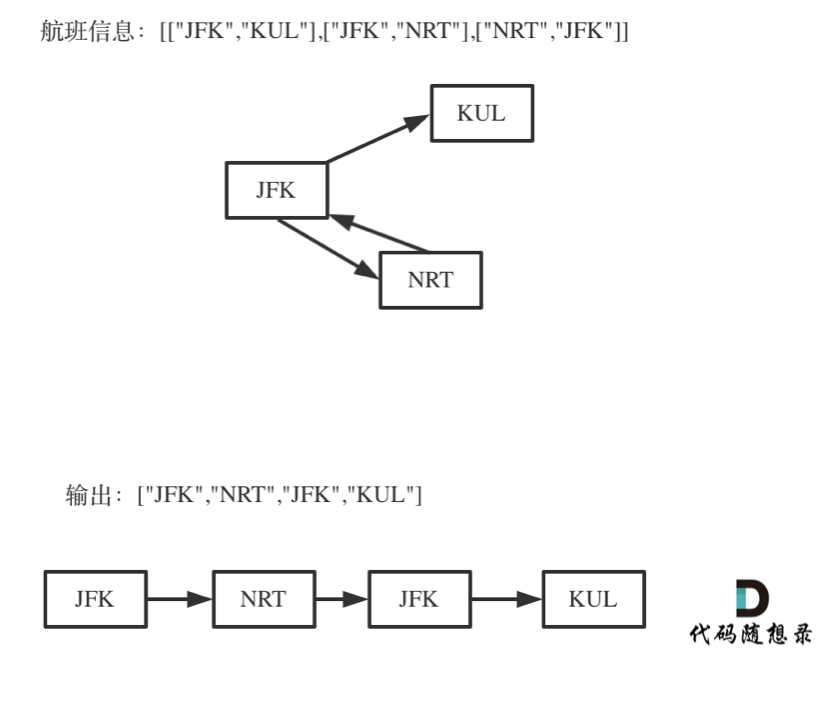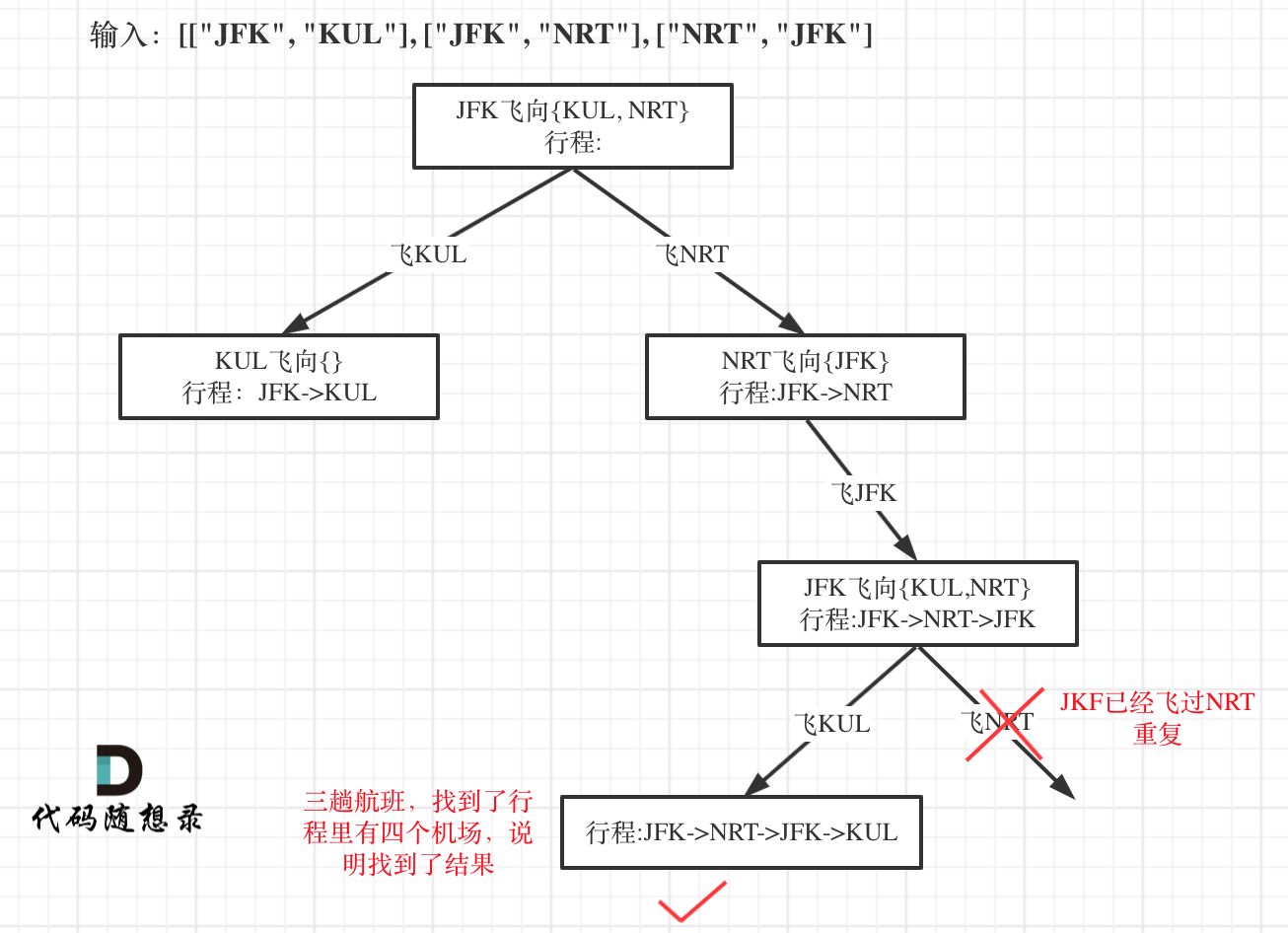## # 332.重新安排行程

• 如果存在多种有效的行程，请你按字符自然排序返回最小的行程组合。例如，行程 ["JFK", "LGA"] 与 ["JFK", "LGB"] 相比就更小，排序更靠前
• 所有的机场都用三个大写字母表示（机场代码）。
• 假定所有机票至少存在一种合理的行程。
• 所有的机票必须都用一次 且 只能用一次。

## # 思路

1. 一个行程中，如果航班处理不好容易变成一个圈，成为死循环
2. 有多种解法，字母序靠前排在前面，让很多同学望而退步，如何该记录映射关系呢 ？
3. 使用回溯法（也可以说深搜） 的话，那么终止条件是什么呢？
4. 搜索的过程中，如何遍历一个机场所对应的所有机场。

## # 如何理解死循环## # 该记录映射关系

`unordered_map<string, multiset<string>> targets``unordered_map<出发机场, 到达机场的集合> targets` `unordered_map<string, map<string, int>> targets``unordered_map<出发机场, map<到达机场, 航班次数>> targets`

## # 回溯法

``````void backtracking(参数) {
if (终止条件) {
存放结果;
return;
}

for (选择：本层集合中元素（树中节点孩子的数量就是集合的大小）) {
处理节点;
backtracking(路径，选择列表); // 递归
回溯，撤销处理结果
}
}
``````
1
2
3
4
5
6
7
8
9
10
11
12• 递归函数参数

``````// unordered_map<出发机场, map<到达机场, 航班次数>> targets
unordered_map<string, map<string, int>> targets;
bool backtracking(int ticketNum, vector<string>& result) {
``````
1
2
3``````for (const vector<string>& vec : tickets) {
targets[vec][vec]++; // 记录映射关系
}
result.push_back("JFK"); // 起始机场
``````
1
2
3
4
• 递归终止条件

``````if (result.size() == ticketNum + 1) {
return true;
}
``````
1
2
3

• 单层搜索的逻辑

``````for (pair<const string, int>& target : targets[result[result.size() - 1]]) {
if (target.second > 0 ) { // 记录到达机场是否飞过了
result.push_back(target.first);
target.second--;
if (backtracking(ticketNum, result)) return true;
result.pop_back();
target.second++;
}
}
``````
1
2
3
4
5
6
7
8
9

``````class Solution {
private:
// unordered_map<出发机场, map<到达机场, 航班次数>> targets
unordered_map<string, map<string, int>> targets;
bool backtracking(int ticketNum, vector<string>& result) {
if (result.size() == ticketNum + 1) {
return true;
}
for (pair<const string, int>& target : targets[result[result.size() - 1]]) {
if (target.second > 0 ) { // 记录到达机场是否飞过了
result.push_back(target.first);
target.second--;
if (backtracking(ticketNum, result)) return true;
result.pop_back();
target.second++;
}
}
return false;
}
public:
vector<string> findItinerary(vector<vector<string>>& tickets) {
targets.clear();
vector<string> result;
for (const vector<string>& vec : tickets) {
targets[vec][vec]++; // 记录映射关系
}
result.push_back("JFK"); // 起始机场
backtracking(tickets.size(), result);
return result;
}
};
``````
1
2
3
4
5
6
7
8
9
10
11
12
13
14
15
16
17
18
19
20
21
22
23
24
25
26
27
28
29
30
31

``````for (pair<const string, int>& target : targets[result[result.size() - 1]])
``````
1

pair里要有const，因为map中的key是不可修改的，所以是`pair<const string, int>`

``````for (pair<string, int>target : targets[result[result.size() - 1]])
``````
1

## # 其他语言版本

java 版本：

``````class Solution {
private Deque<String> res;
private Map<String, Map<String, Integer>> map;

private boolean backTracking(int ticketNum){
if(res.size() == ticketNum + 1){
return true;
}
String last = res.getLast();
if(map.containsKey(last)){//防止出现null
for(Map.Entry<String, Integer> target : map.get(last).entrySet()){
int count = target.getValue();
if(count > 0){
target.setValue(count - 1);
if(backTracking(ticketNum)) return true;
res.removeLast();
target.setValue(count);
}
}
}
return false;
}

public List<String> findItinerary(List<List<String>> tickets) {
map = new HashMap<String, Map<String, Integer>>();
for(List<String> t : tickets){
Map<String, Integer> temp;
if(map.containsKey(t.get(0))){
temp = map.get(t.get(0));
temp.put(t.get(1), temp.getOrDefault(t.get(1), 0) + 1);
}else{
temp = new TreeMap<>();//升序Map
temp.put(t.get(1), 1);
}
map.put(t.get(0), temp);

}
backTracking(tickets.size());
return new ArrayList<>(res);
}
}
``````
1
2
3
4
5
6
7
8
9
10
11
12
13
14
15
16
17
18
19
20
21
22
23
24
25
26
27
28
29
30
31
32
33
34
35
36
37
38
39
40
41
42
43
44

python：

``````class Solution:
def findItinerary(self, tickets: List[List[str]]) -> List[str]:
# defaultdic(list) 是为了方便直接append
tickets_dict = defaultdict(list)
for item in tickets:
tickets_dict[item].append(item)
'''
tickets_dict里面的内容是这样的
{'JFK': ['SFO', 'ATL'], 'SFO': ['ATL'], 'ATL': ['JFK', 'SFO']})
'''
path = ["JFK"]
def backtracking(start_point):
# 终止条件
if len(path) == len(tickets) + 1:
return True
tickets_dict[start_point].sort()
for _ in tickets_dict[start_point]:
#必须及时删除，避免出现死循环
end_point = tickets_dict[start_point].pop(0)
path.append(end_point)
# 只要找到一个就可以返回了
if backtracking(end_point):
return True
path.pop()
tickets_dict[start_point].append(end_point)

backtracking("JFK")
return path
``````
1
2
3
4
5
6
7
8
9
10
11
12
13
14
15
16
17
18
19
20
21
22
23
24
25
26
27
28

C语言版本：

``````char **result;
bool *used;
int g_found;

int cmp(const void *str1, const void *str2)
{
const char **tmp1 = *(char**)str1;
const char **tmp2 = *(char**)str2;
int ret = strcmp(tmp1, tmp2);
if (ret == 0) {
return strcmp(tmp1, tmp2);
}
return ret;
}

void backtracting(char *** tickets, int ticketsSize, int* returnSize, char *start, char **result, bool *used)
{
if (*returnSize == ticketsSize + 1) {
g_found = 1;
return;
}
for (int i = 0; i < ticketsSize; i++) {
if ((used[i] == false) && (strcmp(start, tickets[i]) == 0)) {
result[*returnSize] = (char*)malloc(sizeof(char) * 4);
memcpy(result[*returnSize], tickets[i], sizeof(char) * 4);
(*returnSize)++;
used[i] = true;
/*if ((*returnSize) == ticketsSize + 1) {
return;
}*/
backtracting(tickets, ticketsSize, returnSize, tickets[i], result, used);
if (g_found) {
return;
}
(*returnSize)--;
used[i] = false;
}
}
return;
}

char ** findItinerary(char *** tickets, int ticketsSize, int* ticketsColSize, int* returnSize){
if (tickets == NULL || ticketsSize <= 0) {
return NULL;
}
result = malloc(sizeof(char*) * (ticketsSize + 1));
used = malloc(sizeof(bool) * ticketsSize);
memset(used, false, sizeof(bool) * ticketsSize);
result = malloc(sizeof(char) * 4);
memcpy(result, "JFK", sizeof(char) * 4);
g_found = 0;
*returnSize = 1;
qsort(tickets, ticketsSize, sizeof(tickets), cmp);
backtracting(tickets, ticketsSize, returnSize, "JFK", result, used);
*returnSize = ticketsSize + 1;
return result;
}
``````
1
2
3
4
5
6
7
8
9
10
11
12
13
14
15
16
17
18
19
20
21
22
23
24
25
26
27
28
29
30
31
32
33
34
35
36
37
38
39
40
41
42
43
44
45
46
47
48
49
50
51
52
53
54
55
56
57

Javascript:

``````
var findItinerary = function(tickets) {
let result = ['JFK']
let map = {}

for (const tickt of tickets) {
const [from, to] = tickt
if (!map[from]) {
map[from] = []
}
map[from].push(to)
}

for (const city in map) {
// 对到达城市列表排序
map[city].sort()
}
function backtracing() {
if (result.length === tickets.length + 1) {
return true
}
if (!map[result[result.length - 1]] || !map[result[result.length - 1]].length) {
return false
}
for(let i = 0 ; i <  map[result[result.length - 1]].length; i++) {
let city = map[result[result.length - 1]][i]
// 删除已走过航线，防止死循环
map[result[result.length - 1]].splice(i, 1)
result.push(city)
if (backtracing()) {
return true
}
result.pop()
map[result[result.length - 1]].splice(i, 0, city)
}
}
backtracing()
return result
};

``````
1
2
3
4
5
6
7
8
9
10
11
12
13
14
15
16
17
18
19
20
21
22
23
24
25
26
27
28
29
30
31
32
33
34
35
36
37
38
39
40